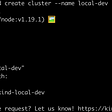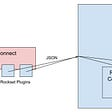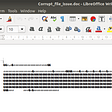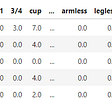# Bit Manipulation

It is easy to know if we only have one element that is unique, and the rest are pairs, we can xor all the numbers to get a unique number.

If we have two numbers only appear once, we can try to separate those two numbers into two groups and do the similar operations. We have the following observations:

1. if two numbers are different, at least one bit will be different.
2. If two numbers are the same, all bits are the same.

From above two observations, suppose we have

[a, b, c1, c2, d1, d2, e1, e2] where a, b, c*, d*, e* are different numbers. c1==c2, d1==d2, e1==e2.

we can divide the number into two groups by using the different bit of a and b.

c1, c2 will have to pick up one group to join. Similar to d1, d2 and e1, e2.

We can use the following operation to get the rightmost 1 for a number:

`diff &= (~diff + 1);`

We can also use diff &= -diff to achieve the same result, however, it is not that obvious. It is based on Two’s complement representation in computer science.

`class Solution{public:    vector<int> singleNumber(vector<int>& nums)     {        auto diff = 0; for (auto num : nums) diff ^= num;        diff &= (~diff + 1);        vector<int> ret(2, 0);        for (auto num : nums)        {            if (num&diff) ret ^= num;            else ret ^= num;        }        return ret;    }};`

# 342. Power of Four

`#define LSOne(S) ((S) & -(S))class Solution {public:    bool isPowerOfFour(int num) {        return (num > 0 &&                 __builtin_popcount(num) == 1 &&                 __builtin_ctz(LSOne(num)) % 2 == 0);    }};`

A more concise one can be found here.

`class Solution {public:    bool isPowerOfFour(int num) {        return (num > 0 &&                 __builtin_popcount(num) == 1 &&                 __builtin_ctz(num) % 2 == 0);    }};`

--

--

--

## More from Jimmy Shen

Data Scientist/MLE/SWE @takemobi

Love podcasts or audiobooks? Learn on the go with our new app.

## Upgrading JetDirect firmware on an old LaserJet 2100 printer## Run a Django project in AWS EC2## Local kubernetes with kind, helm and a sample service## The Ultimate Guide to Electronic Document Management Systems (EDMS)## The Kafka Connect Plugin for Rockset and How It Works## Advice for Less Harmful Singletons## Jimmy Shen

Data Scientist/MLE/SWE @takemobi

## Communication-Efficient SGD Algorithms## Algorithm for Google Search Engine : PageRank Algorithm## Machine Learning Algorithms## The very first and naive information retrieval system